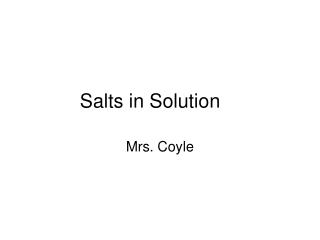DownloadDownload PresentationSalts in Solution

# Salts in Solution

Download Presentation## Salts in Solution

- - - - - - - - - - - - - - - - - - - - - - - - - - - E N D - - - - - - - - - - - - - - - - - - - - - - - - - - -
##### Presentation Transcript

1. Salts in Solution Mrs. Coyle

2. Solutions of Salts -Strong Acids and Strong Bases • Produce a neutral solution (pH=7) • Example: HCl + NaOH  NaCl + H2O Strong AcidStrong Base Neutral Solution

3. Solutions of Salts -Strong Acids and Weak Bases • Produce an acidic solution (pH<7) • Example: HCl + NH3 NH 4Cl + H2O Strong AcidWeak Base Acidic Solution

4. Solutions of Salts -Weak Acids and Strong Bases • Produce a basic solution (pH>7) • Example: H2C O3 + KOH  K2C O3 + H2O Weak AcidStrong Base Basic Solution

5. Solutions of Salts -Weak Acids and Weak Bases • The pH of their salt’s solution depends on their relative strength.

6. Summary Strong Acid + Strong Base Neutral Solution Strong Acid + Weak BaseAcidic Solution Weak Acid + Strong BaseBasic Solution

7. Why does this happen? • SaltHydrolysis • Ions of the dissociated salt, remove or donate H+ , to the solution.

8. Example NH4Cl (aq)  NH 4+ (aq) + Cl- (aq) NH 4+ (aq) + H2O (l)  NH3 (aq) + H3O+(aq) The H3O+ concentration is greater than that of OH- , so the solution is acidic.

9. Titration of Weak Acid with Strong Base Equivalence Point is pH=8.7 Note: Equivalence point of pH=7 for HCl(strong) and NaOH(strong) http://www.chem.ubc.ca/courseware/pH/section14/ph2.jpg

10. Buffers • Solution in which the pH remains relatively constant when a small amount of acid or base is added to the solution. • They are generally a weak acid and its salt or a weak base and its salt.

11. Examples of Buffers • H2CO3 and the salt of HCO3- (in human blood) • NH3 and the salt of NH4+

12. Indicators